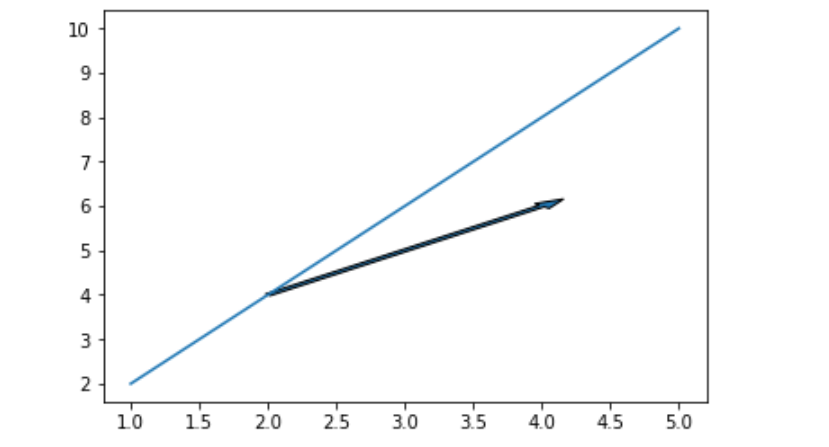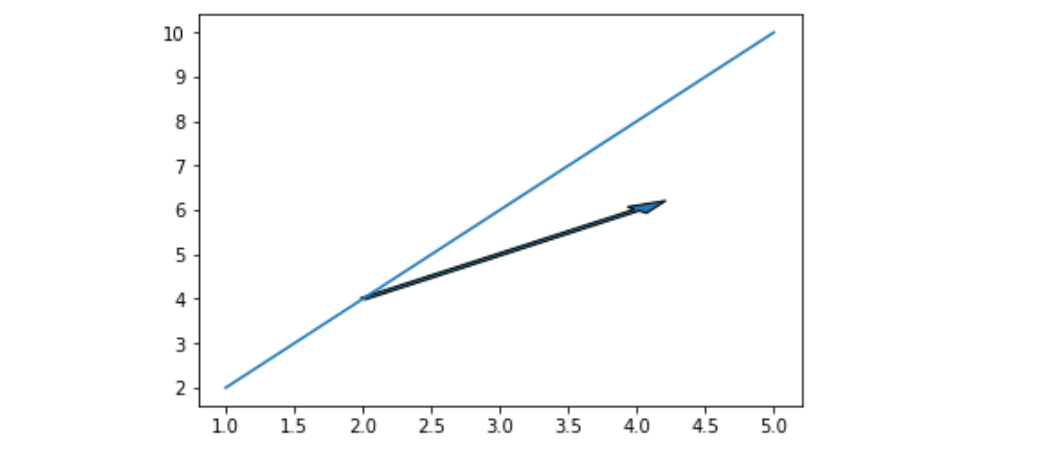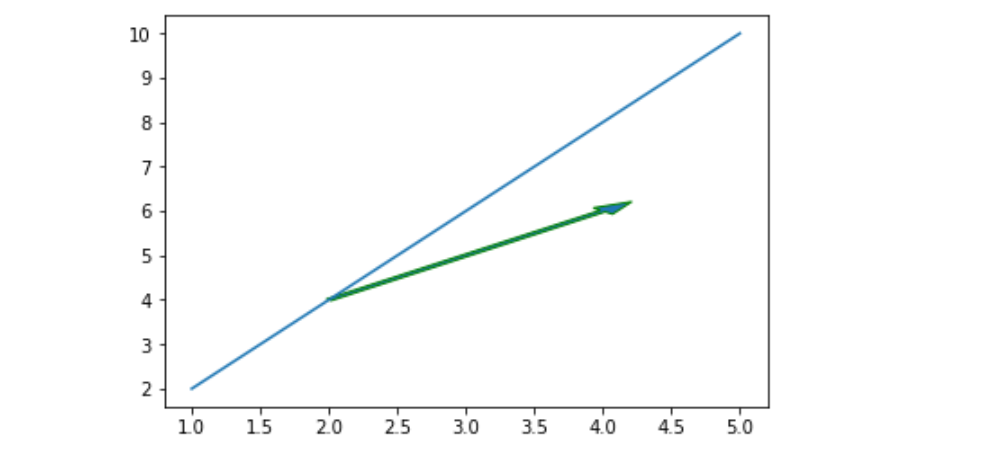Related Articles
matplotlib.pyplot.arrow() in Python
• Last Updated : 12 Apr, 2020

Matplotlib is a very powerful plotting library useful for those working with Python and NumPy. And for making statistical interference, it becomes very necessary to visualize our data and Matplotlib is the tool that can be very helpful for this purpose. It provides MATLAB like interface only difference is that it uses Python and is open source.

## matplotlib.pyplot.arrow()

This function adds the arrow to the graph based on the coordinates passed to it.

Syntax: matplotlib.pyplot.arrow(x, y, dx, dy, **kwargs)

Parameters:
x, y: The x and y coordinates of the arrow base.
dx, dy: The length of the arrow along x and y direction.
**kwargs: Optional arguments that helps in adding properties to arrow, like
adding color to arrow, changing width of arrow

Example #1

 `import` `matplotlib.pyplot as plt`` ` ` ` `# Initilaizing values ``# of x and y``x ``=``[``1``, ``2``, ``3``, ``4``, ``5``]``y ``=``[``2``, ``4``, ``6``, ``8``, ``10``]`` ` `# Plotting the graph``plt.plot(x, y)`` ` `# Adding an arrow to graph starting ``# from the base (2, 4) and with the ``# length of 2 units from both x and y``# And setting the width of arrow for``# better visualization``plt.arrow(``2``, ``4``, ``2``, ``2``, width ``=` `0.05``)`` ` `# Showing the graph``plt.show()`

Output:Example 2#

 `import` `matplotlib.pyplot as plt`` ` ` ` `x ``=``[``1``, ``2``, ``3``, ``4``, ``5``]``y ``=``[``2``, ``4``, ``6``, ``8``, ``10``]`` ` `plt.plot(x, y)`` ` `# Increasing head_width of``# the arrow by setting ``# head_width parameter``plt.arrow(``2``, ``4``, ``2``, ``2``, ``          ``head_width ``=` `0.2``, ``          ``width ``=` `0.05``)`` ` `plt.show()`

Output:Example #3

 `import` `matplotlib.pyplot as plt`` ` ` ` `x ``=``[``1``, ``2``, ``3``, ``4``, ``5``]``y ``=``[``2``, ``4``, ``6``, ``8``, ``10``]``plt.plot(x, y)`` ` `# changing the edge color``# to green``plt.arrow(``2``, ``4``, ``2``, ``2``, ``          ``head_width ``=` `0.2``,``          ``width ``=` `0.05``,``          ``ec ``=``'green'``)`` ` `plt.show()`

Output:Attention geek! Strengthen your foundations with the Python Programming Foundation Course and learn the basics.

To begin with, your interview preparations Enhance your Data Structures concepts with the Python DS Course.

My Personal Notes arrow_drop_up